# 【Python基础】第十一课：处理缺失值

## 缺失值的产生，缺失值的表示，检测缺失值，舍弃缺失值，填补缺失值

Posted by x-jeff on February 23, 2020

# 1.侦测缺失值

## 1.1.缺失值的产生

1. 机械缺失：例如机械故障，导致数据无法被完整保存。
2. 人为缺失：例如受访者拒绝透漏部分信息。

## 1.2.缺失值的表示

1
2
import numpy as np
print(np.nan)#nan


## 1.3.检测缺失值

1
2
3
4
5
6
7
import numpy as np
import pandas as pd
#构建一组含有缺失值的数据
df=pd.DataFrame([["Tim","M",24,169,100],["Jack","M",np.nan,177,140],["Jessy","F",21,162,np.nan],["Mary","F",23,159,87]])
#赋予列名
df.columns=["Name","Gender","Age","Height","Weight"]
print(df)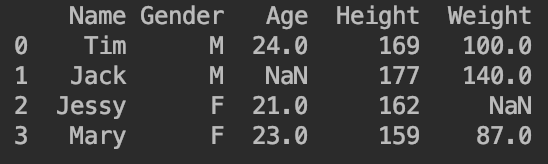👉检查是否存在缺失值：.isnull().values.any()

1
2
3
4
5
6
7
#检查第1行是否存在缺失值
print(df.loc.isnull().values.any())#返回False说明无缺失值
print(df[0:1].isnull().values.any())#另一种表达方式，也是检查第1行是否有缺失值
#检查第3列是否存在缺失值
print(df["Age"].isnull().values.any())#返回True说明存在缺失值
#判断整个DataFrame中是否存在缺失值
print(df.isnull().values.any())#返回True说明DataFrame中存在缺失值


👉输出缺失值的具体位置：.isnull().notnull()

1
2
3
4
5
6
7
8
9
10
11
#判断第4行缺失值的具体位置
print(df.loc.isnull())#False为非缺失值，True为缺失值
print(df.loc.notnull())#False为缺失值，True为非缺失值
#判断第5列缺失值的具体位置
print(df["Weight"].isnull())
print(df["Weight"].notnull())
#判断整个DataFrame中是否存在缺失值
print(df.isnull())
print(df.notnull())
#同时检查所有列是否存在缺失值
print(df.isnull().any())


print(df.isnull())为例，其输出为：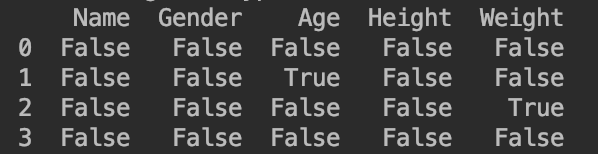print(df.isnull().any())输出为：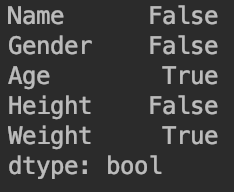👉统计缺失值的数量：.isnull().sum()

1
2
3
4
5
6
7
#统计第2行缺失值的数量
print(df.loc.isnull().sum())#output:1
#统计第3列缺失值的数量
print(df["Age"].isnull().sum())#output:1
#整个DataFrame缺失值的数量
print(df.isnull().sum())#按列统计
print(df.isnull().sum().sum())#总计;output:2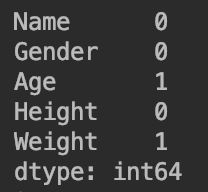# 2.处理缺失值

## 2.1.舍弃缺失值

1
2
3
4
5
6
import pandas as pd
import numpy as np
df=pd.DataFrame([["Tim","M",24,169,100],["Jack","M",np.nan,177,np.nan],["Jessy","F",21,162,np.nan],["Mary","F",23,159,87]])
df.columns=["Name","Gender","Age","Height","Weight"]
df["Salary"]=np.nan
print(df)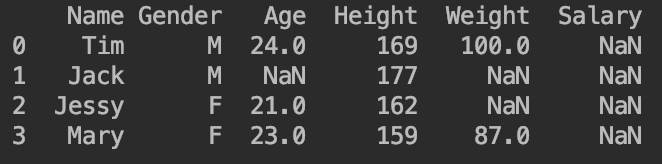👉舍弃含有缺失值(缺失值数量>0)的行/列：

1
2
3
4
#舍弃含有缺失值的行
print(df.dropna(axis=0,how="any"))#默认参数
#舍弃含有缺失值的列
print(df.dropna(axis=1,how="any"))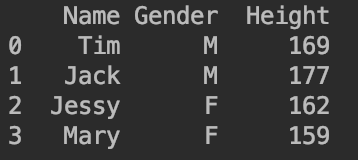👉舍弃全部为缺失值的行/列：

1
2
#以列为例：axis=1
print(df.dropna(axis=1,how="all"))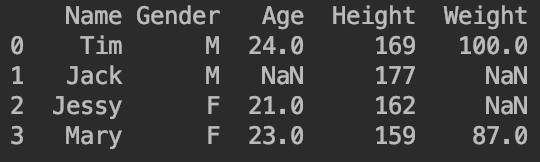👉仅保留非缺失值数量大于等于一定阈值的行/列：

1
2
#以行为例：axis=0
print(df.dropna(axis=0,thresh=4))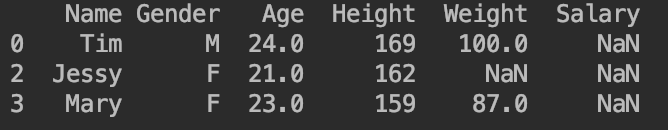## 2.2.填补缺失值

1. 使用叙述性统计填补缺失值。
2. 使用内插法填补缺失值。

### 2.2.1.叙述性统计填补缺失值

#### 2.2.1.1.用某个具体数值填补缺失值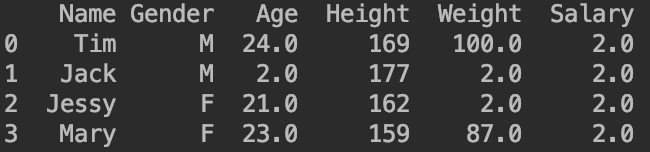#### 2.2.1.2.用平均值填补缺失值

1
2
df["Age"].fillna(df["Age"].mean(),inplace=True)
print(df)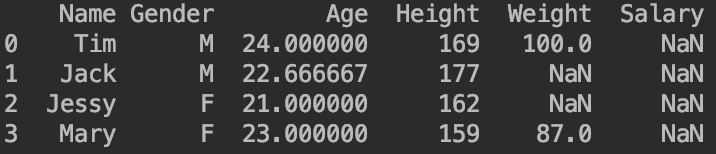#### 2.2.1.3.用分层平均值填补缺失值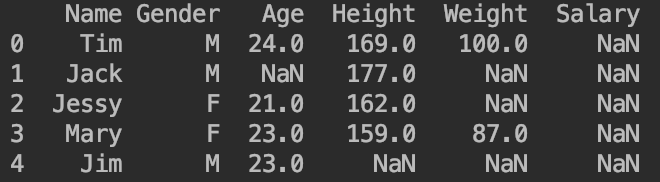1
2
3
4
5
6
#方式一
df["Age"].fillna(df["Age"].groupby(df["Gender"]).transform("mean"),inplace=True)
print(df)
#方式二
df["Age"].fillna(df.groupby("Gender")["Age"].transform("mean"),inplace=True)
print(df)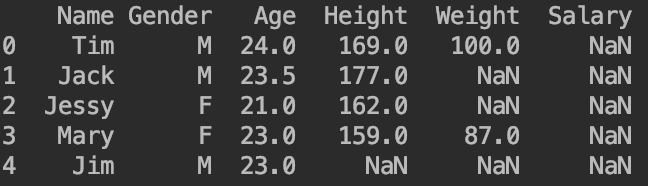groupbytransform的详细用法见第3部分。

#### 2.2.1.4.向前/后填值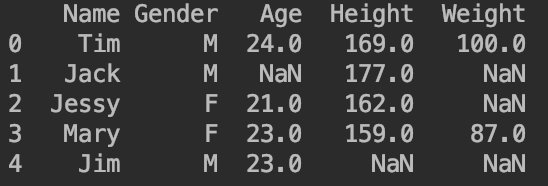👉向后填补缺失值：第二行参照第一行，第三行参照第二行，以此类推。

1
2
#向后填补缺失值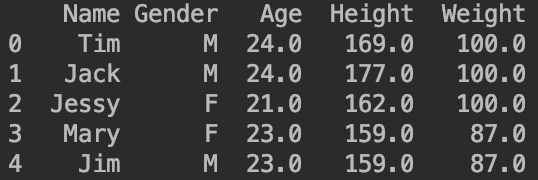👉向前填补缺失值：倒数第二行参照最后一行，倒数第三行参照倒数第二行，以此类推。

1
2
#向前填补缺失值
df.fillna(method="bfill",inplace=True)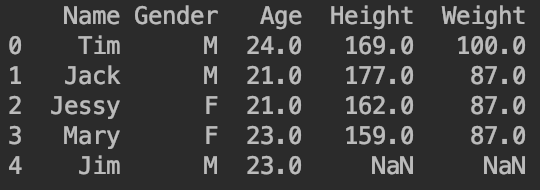1
2
#在向前填补缺失值时，只填补一行
df.fillna(method="bfill",inplace=True,limit=1)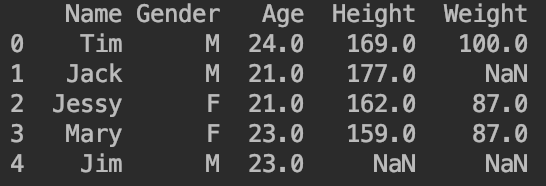### 2.2.2.内插法填补缺失值

1
2
3
df=pd.DataFrame([[1,870],[2,900],[np.nan,np.nan],[4,950],[5,1000],[6,1200]])
df.columns=["Time","Value"]
print(df)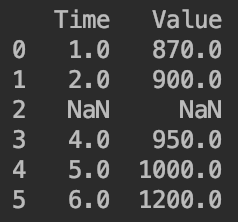1
2
#使用内插法填补缺失值
print(df.interpolate())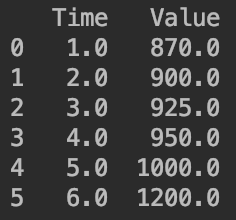# 3.groupby和transform

1
2
3
np.random.seed(1)
df=pd.DataFrame({"key1":list('aabba'),"key2":["one","two","one","two","one"],"data1":np.random.randn(5),"data2":np.random.randn(5)})
print(df)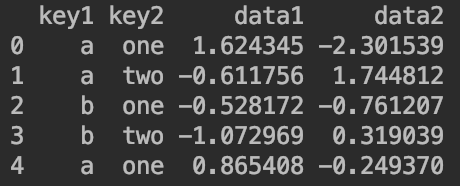1
2
grouped=df["data1"].groupby(df["key1"])
print(grouped.mean())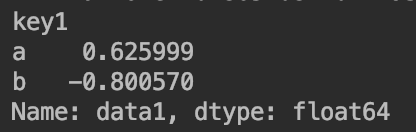1. 方式一：df["data1"]=df["data1"].groupby(df["key1"]).transform("mean")
2. 方式二：df["data1"]=df.groupby("key1")["data1"].transform("mean")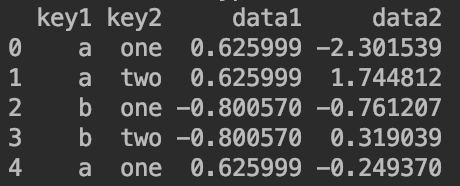# 4.代码地址